# Audio I/O and Pre-Processing with torchaudio

Note: This is an R port of the official tutorial available here. All credits goes to Vincent Quenneville-Bélair.

{torch} is an open source deep learning platform that provides a seamless path from research prototyping to production deployment with GPU support.

Significant effort in solving machine learning problems goes into data preparation. torchaudio leverages torch’s GPU support, and provides many tools to make data loading easy and more readable. In this tutorial, we will see how to load and preprocess data from a simple dataset.

library(torchaudio)
library(viridis)

# Opening a file

torchaudio also supports loading sound files in the wav and mp3 format. We call waveform the resulting raw audio signal.

url = "https://pytorch.org/tutorials/_static/img/steam-train-whistle-daniel_simon-converted-from-mp3.wav"
filename = tempfile(fileext = ".wav")
r = httr::GET(url, httr::write_disk(filename, overwrite = TRUE))

waveform = waveform_and_sample_rate[]
sample_rate = waveform_and_sample_rate[]

paste("Shape of waveform: ", paste(dim(waveform), collapse = " "))
#>  "Shape of waveform:  2 276858"
paste("Sample rate of waveform: ", sample_rate)
#>  "Sample rate of waveform:  44100"

plot(waveform, col = "royalblue", type = "l")
lines(waveform, col = "orange")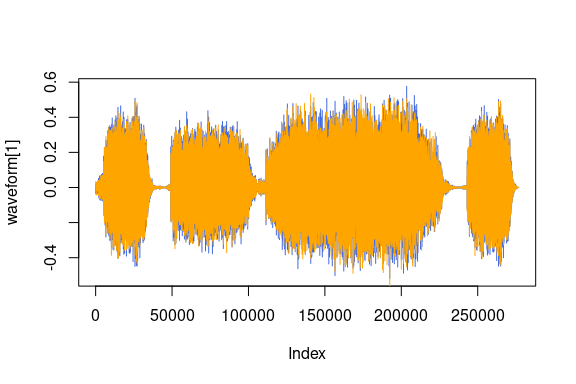Package {tuneR} is the only backend implemented yet.

## Transformations

torchaudio supports a growing list of transformations.

• Resample: Resample waveform to a different sample rate.
• Spectrogram: Create a spectrogram from a waveform.
• GriffinLim: Compute waveform from a linear scale magnitude spectrogram using the Griffin-Lim transformation.
• ComputeDeltas: Compute delta coefficients of a tensor, usually a spectrogram.
• ComplexNorm: Compute the norm of a complex tensor.
• MelScale: This turns a normal STFT into a Mel-frequency STFT, using a conversion matrix.
• AmplitudeToDB: This turns a spectrogram from the power/amplitude scale to the decibel scale.
• MFCC: Create the Mel-frequency cepstrum coefficients from a waveform.
• MelSpectrogram: Create MEL Spectrograms from a waveform using the STFT function in Torch.
• MuLawEncoding: Encode waveform based on mu-law companding.
• MuLawDecoding: Decode mu-law encoded waveform.
• TimeStretch: Stretch a spectrogram in time without modifying pitch for a given rate.
• FrequencyMasking: Apply masking to a spectrogram in the frequency domain.
• TimeMasking: Apply masking to a spectrogram in the time domain.

Each transform supports batching: you can perform a transform on a single raw audio signal or spectrogram, or many of the same shape.

Since all transforms are torch::nn_modules, they can be used as part of a neural network at any point.

To start, we can look at the log of the spectrogram on a log scale.

specgram <- transform_spectrogram()(waveform)

paste("Shape of spectrogram: ", paste(dim(specgram), collapse = " "))
#>  "Shape of spectrogram:  2 201 1385"

specgram_as_array <- as.array(specgram$log2()$t())
image(specgram_as_array[,ncol(specgram_as_array):1], col = viridis(n = 257,  option = "magma"))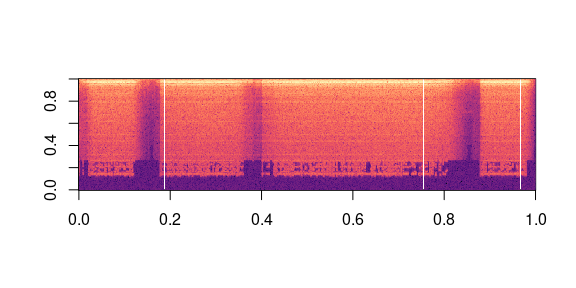Or we can look at the Mel Spectrogram on a log scale.

specgram <- transform_mel_spectrogram()(waveform)

paste("Shape of spectrogram: ", paste(dim(specgram), collapse = " "))
#>  "Shape of spectrogram:  2 128 1385"

specgram_as_array <- as.array(specgram$log2()$t())
image(specgram_as_array[,ncol(specgram_as_array):1], col = viridis(n = 257,  option = "magma"))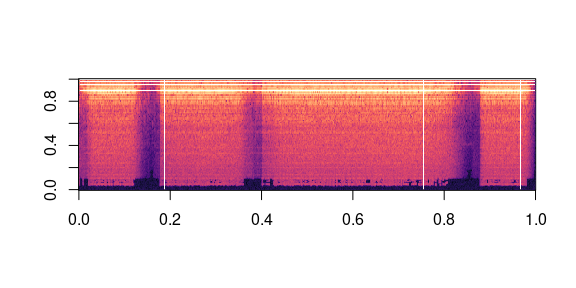We can resample the waveform, one channel at a time.

new_sample_rate <- sample_rate/10

# Since Resample applies to a single channel, we resample first channel here
channel <- 1
transformed <- transform_resample(sample_rate, new_sample_rate)(waveform[channel, ]$view(c(1,-1))) paste("Shape of transformed waveform: ", paste(dim(transformed), collapse = " ")) #>  "Shape of transformed waveform: 1 27686" plot(transformed, col = "royalblue", type = "l")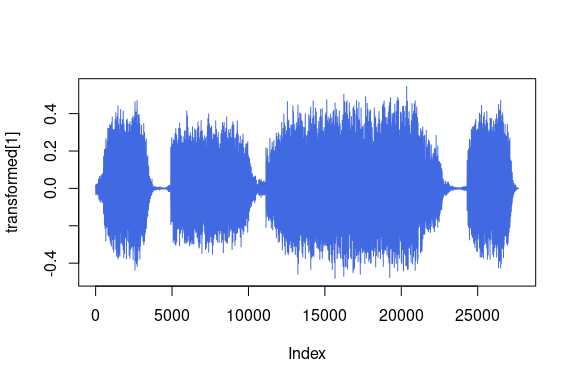As another example of transformations, we can encode the signal based on Mu-Law enconding. But to do so, we need the signal to be between -1 and 1. Since the tensor is just a regular PyTorch tensor, we can apply standard operators on it. # Let's check if the tensor is in the interval [-1,1] cat(sprintf("Min of waveform: %f \nMax of waveform: %f \nMean of waveform: %f", as.numeric(waveform$min()), as.numeric(waveform$max()), as.numeric(waveform$mean())))
#> Min of waveform: -0.572845
#> Max of waveform: 0.575958
#> Mean of waveform: 0.000093

Since the waveform is already between -1 and 1, we do not need to normalize it.

normalize <- function(tensor) {
# Subtract the mean, and scale to the interval [-1,1]
tensor_minusmean <- tensor - tensor.mean()
return(tensor_minusmean/tensor_minusmean$abs()$max())
}

# Let's normalize to the full interval [-1,1]
# waveform = normalize(waveform)

Let’s apply encode the waveform.

transformed <- transform_mu_law_encoding()(waveform)

paste("Shape of transformed waveform: ", paste(dim(transformed), collapse = " "))
#>  "Shape of transformed waveform:  2 276858"

plot(transformed, col = "royalblue", type = "l")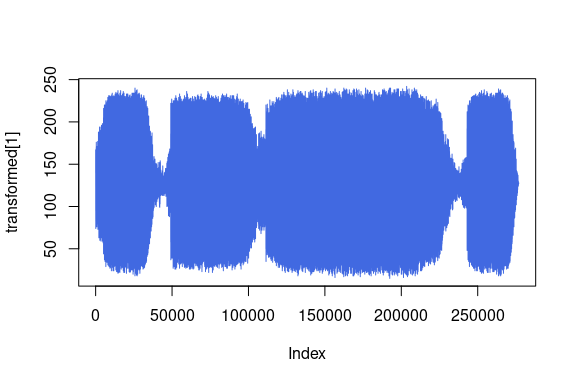And now decode.

reconstructed <- transform_mu_law_decoding()(transformed)

paste("Shape of recovered waveform: ", paste(dim(reconstructed), collapse = " "))
#>  "Shape of recovered waveform:  2 276858"

plot(reconstructed, col = "royalblue", type = "l")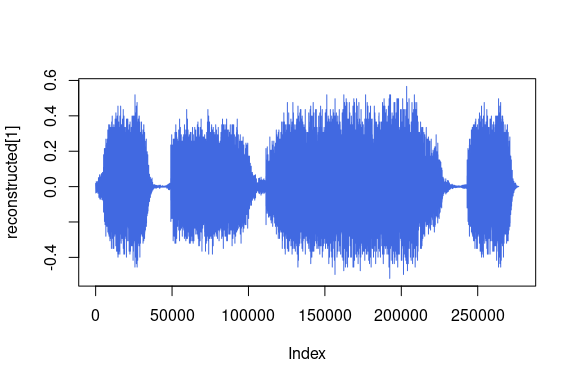We can finally compare the original waveform with its reconstructed version.

# Compute median relative difference
err <- as.numeric(((waveform - reconstructed)$abs() / waveform$abs())$median()) paste("Median relative difference between original and MuLaw reconstucted signals:", scales::percent(err, accuracy = 0.01)) #>  "Median relative difference between original and MuLaw reconstucted signals: 1.28%" # Functional The transformations seen above rely on lower level stateless functions for their computations. These functions are identified by torchaudio::functional_* prefix. • istft: Inverse short time Fourier Transform. • gain: Applies amplification or attenuation to the whole waveform. • dither: Increases the perceived dynamic range of audio stored at a particular bit-depth. • compute_deltas: Compute delta coefficients of a tensor. • equalizer_biquad: Design biquad peaking equalizer filter and perform filtering. • lowpass_biquad: Design biquad lowpass filter and perform filtering. • highpass_biquad:Design biquad highpass filter and perform filtering. For example, let’s try the functional_mu_law_encoding: mu_law_encoding_waveform <- functional_mu_law_encoding(waveform, quantization_channels = 256) paste("Shape of transformed waveform: ", paste(dim(mu_law_encoding_waveform), collapse = " ")) #>  "Shape of transformed waveform: 2 276858" plot(mu_law_encoding_waveform, col = "royalblue", type = "l")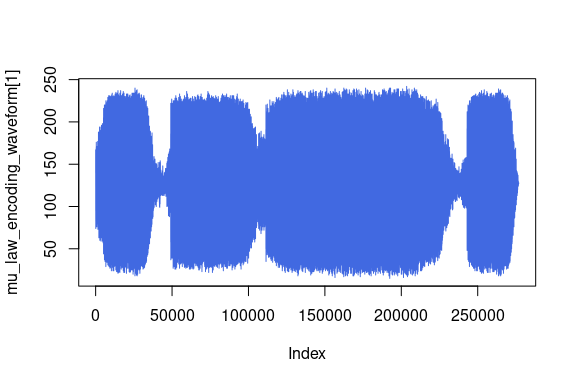You can see how the output from functional_mu_law_encoding is the same as the output from transforms_mu_law_encoding. Now let’s experiment with a few of the other functionals and visualize their output. Taking our spectogram, we can compute it’s deltas: computed <- functional_compute_deltas(specgram$contiguous(), win_length=3)

paste("Shape of computed deltas: ", paste(dim(computed), collapse = " "))
#>  "Shape of computed deltas:  2 128 1385"

computed_as_array <- as.array(computed\$t())
image(computed_as_array[,ncol(computed_as_array):1], col = viridis(n = 257,  option = "magma"))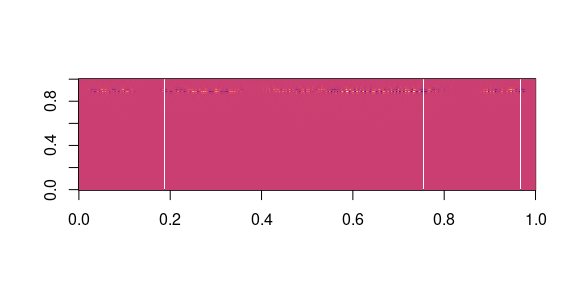We can take the original waveform and apply different effects to it.

gain_waveform <- as.numeric(functional_gain(waveform, gain_db=5.0))
cat(sprintf("Min of gain_waveform: %f\nMax of gain_waveform: %f\nMean of gain_waveform: %f", min(gain_waveform), max(gain_waveform), mean(gain_waveform)))
#> Min of gain_waveform: -1.018679
#> Max of gain_waveform: 1.024215
#> Mean of gain_waveform: 0.000165

dither_waveform <- as.numeric(functional_dither(waveform))
cat(sprintf("Min of dither_waveform: %f\nMax of dither_waveform: %f\nMean of dither_waveform: %f", min(dither_waveform), max(dither_waveform), mean(dither_waveform)))
#> Min of dither_waveform: -0.572784
#> Max of dither_waveform: 0.575928
#> Mean of dither_waveform: 0.000107

Another example of the capabilities in torchaudio::functional_ are applying filters to our waveform. Applying the lowpass biquad filter to our waveform will output a new waveform with the signal of the frequency modified.

lowpass_waveform <- as.array(functional_lowpass_biquad(waveform, sample_rate, cutoff_freq=3000))

cat(sprintf("Min of lowpass_waveform: %f\nMax of lowpass_waveform: %f\nMean of lowpass_waveform: %f", min(lowpass_waveform), max(lowpass_waveform), mean(lowpass_waveform)))
#> Min of lowpass_waveform: -0.559506
#> Max of lowpass_waveform: 0.559501
#> Mean of lowpass_waveform: 0.000093

plot(lowpass_waveform[1,], col = "royalblue", type = "l")
lines(lowpass_waveform[2,], col = "orange")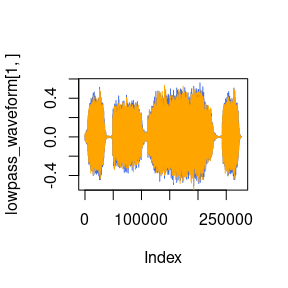We can also visualize a waveform with the highpass biquad filter.

highpass_waveform <- as.array(functional_highpass_biquad(waveform, sample_rate, cutoff_freq=3000))

cat(sprintf("Min of highpass_waveform: %f\nMax of highpass_waveform: %f\nMean of highpass_waveform: %f", min(highpass_waveform), max(highpass_waveform), mean(highpass_waveform)))
#> Min of highpass_waveform: -0.067884
#> Max of highpass_waveform: 0.059494
#> Mean of highpass_waveform: -0.000000

plot(highpass_waveform[1,], col = "royalblue", type = "l")
lines(highpass_waveform[2,], col = "orange")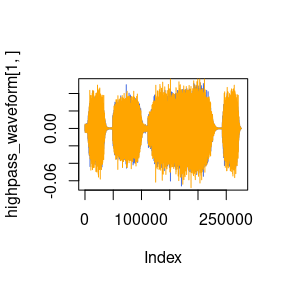# Migrating to torchaudio from Kaldi (Not Implemented Yet)

Users may be familiar with Kaldi, a toolkit for speech recognition. torchaudio will offer compatibility with it in torchaudio::kaldi_* in the future.

# Available Datasets

If you do not want to create your own dataset to train your model, torchaudio offers a unified dataset interface. This interface supports lazy-loading of files to memory, download and extract functions, and datasets to build models.

The datasets torchaudio currently supports are:

• Yesno

• SpeechCommands

• CMUArctics

temp <- tempdir()

# A data point in Yesno is a list (waveform, sample_rate, labels) where labels is a list of integers with 1 for yes and 0 for no.

# Pick data point number 3 to see an example of the the yesno_data:
n <- 3
sample <- yesno_data[n]
sample
#> []
#> torch_tensor
#> Columns 1 to 10-0.0000  0.0000 -0.0000 -0.0000  0.0000  0.0000  0.0000 -0.0000 -0.0000 -0.0000
#>  0.0000  0.0000  0.0000  0.0000  0.0000  0.0000  0.0000  0.0000  0.0000  0.0000
#>
#> Columns 11 to 20-0.0000  0.0000  0.0001  0.0000  0.0000  0.0000  0.0001  0.0000  0.0000  0.0000
#>  0.0000  0.0000  0.0000  0.0000  0.0000  0.0000  0.0000  0.0000  0.0000  0.0000
#>
#> Columns 21 to 30 0.0001  0.0000  0.0000  0.0000  0.0000  0.0000 -0.0000  0.0000 -0.0000  0.0000
#>  0.0000  0.0000  0.0000  0.0000  0.0000  0.0000  0.0000  0.0000  0.0000  0.0000
#>
#> Columns 31 to 40-0.0000  0.0000 -0.0000  0.0000 -0.0000  0.0000  0.0000  0.0000  0.0000  0.0000
#>  0.0000  0.0000  0.0000  0.0000  0.0000  0.0000  0.0000  0.0000  0.0000  0.0000
#>
#> Columns 41 to 50 0.0000 -0.0000 -0.0000 -0.0000  0.0000  0.0000  0.0000  0.0000  0.0000 -0.0001
#>  0.0000  0.0000  0.0000  0.0000  0.0000  0.0000  0.0000  0.0000  0.0000  0.0000
#>
#> Columns 51 to 60-0.0002 -0.0002 -0.0004 -0.0005 -0.0006 -0.0008 -0.0009 -0.0009 -0.0009 -0.0010
#>  0.0000  0.0000  0.0000  0.0000  0.0000  0.0000  0.0000  0.0000  0.0000  0.0000
#>
#> Columns 61 to 70-0.0011 -0.0012 -0.0013 -0.0012 -0.0014 -0.0015 -0.0014 -0.0014 -0.0014 -0.0016
#>  0.0000  0.0000  0.0000  0.0000  0.0000  0.0000  0.0000  0.0000  0.0000  0.0000
#>
#> Columns 71 to 80-0.0018 -0.0018 -0.0018 -0.0019 -0.0020 -0.0020 -0.0020 -0.0023 -0.0025 -0.0023
#>  0.0000  0.0000  0.0000  0.0000  0.0000  0.0000  0.0000  0.0000  0.0000  0.0000
#>
#> Columns 81 to 90-0.0022 -0.0023 -0.0018 -0.0019 -0.0019 -0.0021 -0.0024 -0.0022 -0.0021 -0.0017
#>  0.0000  0.0000  0.0000  0.0000  0.0000  0.0000  0.0000  0.0000  0.0000  0.0000
#>
#> Columns 91 to 100-0.0014 -0.0015 -0.0018 -0.0019 -0.0020 -0.0019 -0.0015 -0.0013 -0.0013 -0.0010
#>  0.0000  0.0000  0.0000  0.0000  0.0000  0.0000  0.0000  0.0000  0.0000  0.0000
#>
#> ... [the output was truncated (use n=-1 to disable)]
#> [ CPUFloatType{2,48880} ]
#>
#> []
#>  8000
#>
#> []
#>  0 0 0 1 0 1 1 0

plot(sample[], col = "royalblue", type = "l")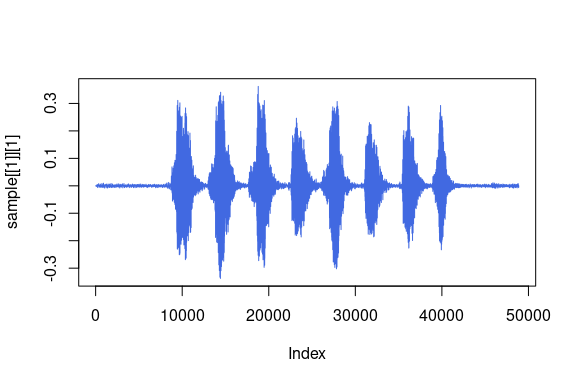Now, whenever you ask for a sound file from the dataset, it is loaded in memory only when you ask for it. Meaning, the dataset only loads and keeps in memory the items that you want and use, saving on memory.

# Conclusion

We used an example raw audio signal, or waveform, to illustrate how to open an audio file using torchaudio, and how to pre-process, transform, and apply functions to such waveform. We also demonstrated built-in datasets to construct our models. Given that torchaudio is built on {torch}, these techniques can be used as building blocks for more advanced audio applications, such as speech recognition, while leveraging GPUs.